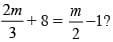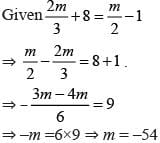# Olympiad Test : Algebra - 1

## 10 Questions MCQ Test Maths Olympiad Class 6 | Olympiad Test : Algebra - 1

Description
Attempt Olympiad Test : Algebra - 1 | 10 questions in 20 minutes | Mock test for Class 6 preparation | Free important questions MCQ to study Maths Olympiad Class 6 for Class 6 Exam | Download free PDF with solutions
QUESTION: 1

### What must be subtracted from a3 – 4a2 + 5a – 6 to obtain a2 – 2a + 1?

Solution:

Here, a3– 4a2 +  5a – 6 – (a2 – 2a + 1)
= a3– 4a2 + 5a – 6 – a2 + 2a – 1
= a3– 5a2 + 7a – 7

QUESTION: 2

### By how much does 1 exceed 2x – 3y – 4 ?

Solution:

Required difference = 1– (2x – 3y – 4)
= 3y – 2x + 5

QUESTION: 3

### How much less than l – 2m + 3n is 2l – 4m – n ?

Solution:

Required difference
= (l – 2m + 3n) – (2l – 4m – n)
= – l + 2m + 4n

QUESTION: 4

If x  = – 2 y = – 1 and z = 3 then what is the value of x2 + y2 – z2

Solution:

Required value
= x2 + y2 – z2
= (–2)2 + (–1)2– (3)2 = 4 + 1 – 9
= 5 – 9 = – 4

QUESTION: 5

What is the co-efficient of m in –7 l2 mn ?

Solution:
QUESTION: 6

If x = 4, y = –1 and z = –2 then what is the value of 2x2 – y2 + 3z2 ?

Solution:

2x2 –y2 + 3z2
= 2(4)2 – (–1)2 + 3(–2)2
= 32 – 1 + 12 = 44 – 1= 43

QUESTION: 7

What must be added to 5x3 – 2x2 + 6x + 7 to make the sum x3 + 3x2 – x + 1 ?

Solution:

x3 + 3x2 – x + 1 – 5x3 + 2x2 – 6x – 7
= –4 x3 + 5x2 – 7x – 6

QUESTION: 8

By how much is 2x – 3y + 4z greater than 2x + 5y – 6z + 2?

Solution:

Required difference
= – (–2x – 5y + 6z – 2)
= – 8y + 10z – 2

QUESTION: 9

What is the simplified value of 2x – [3y –{2x– (y – x)}]?

Solution:

2x –[3y–{2x– (y–x)}]
= 2x –[3y–{2x– y + x)}]
= 2x –[3y–3x + y] = 2x – 4y + 3x = 5x–4y

QUESTION: 10

What is the value of m ifSolution:Use Code STAYHOME200 and get INR 200 additional OFF Use Coupon Code